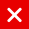# IOTA Tutorial

## Create and Validate a Signature

### HOW AN ADDRESS IS CALCULATED

Before I explain how a signature in a bundle is created and validated, it is important to understand how IOTA addresses are calculated.

In IOTA tutorial 9.1, I have explained in detail how the key, digests and address are created. Here is a short explanation:

•  A key (= private key) is generated using the seed, index number and security level.
• The index number is an integer (0,1,2,.., 9007199254740991) and every address has a corresponding index number.
• The generated key is divided in segments of 81 trytes each.
• Depending on the selected security level (1,2 or 3) you will have (1×27=) 27, (2×27=) 54 or (3×27=) 81 segments. –
• Each key segment is hashed 26 times.
• 27 key segments together forms a keyFragment and each keyFragment is hashed one time to create a digests.
• Depending on the selected security level, 1, 2 or 3 digests are combined together.
• The combined digests are hashed one time to create the address.

### HOW A SIGNATURE IS CREATED

The generated key is divided in segments of 81 trytes each. Depending on the selected security level (1,2 or 3) you will have (1×27=) 27, (2×27=) 54 or (3×27=) 81 segments.

To create a signature:

• Calculate the normalizedBundleHash = normalizedBundle(bundleHash) The bundleHash is explained in IOTA tutorial 15. The normalizedBundleHash is explained in IOTA tutorial 16.
• Convert each tryte in the normalizedBundleHash to its decimal value.
• Apply for each decimal value the following calculation: K = 13 – decimal value.
• Each key segment must be hashed K times.
• Combine the 27 hashed key segments to a signatureFragment. A signatureFragment has (27×81=) 2187 trytes.
• Depending on the selected security level you will have 1, 2 or 3 signatureFragments.
• A transactionObject stores a signatureFragment in the signatureMessageFragment field. This field size is 2187 trytes. Additional transactionObjects are needed to store the remaining signatureFragments.
• Note: signatureMessageFragments is the same as signatureFragments.

### HOW A SIGNATURE IS VALIDATED

To validate a signature in a transaction bundle:

• Extract all corresponding signatureMessageFragments from the bundle. Depending on the selected security level you will have 1, 2 or 3 signatureMessageFragments.
• The combined signatureMessageFragments is called the signature.
• Extract the bundlehash from the bundle. Note: The bundleHash value is the same within the bundle.
• A signature consists of 1,2 or 3 signatureMessageFragments (= signatureFragments), depending on the selected security level.
• Calculate the normalizedBundleHash = normalizedBundle(bundleHash)
• Convert each tryte in the normalizedBundleHash to its decimal value.
• Apply for each decimal value the following calculation: K = 13 + decimal value.
• Each signatureFragment segment must be hashed K times.
• Combine the 27 hashed signatureFragment segments to a keyFragment.
• A keyFragment has (27×81=) 2187 trytes.
• Each keyFragment is hashed one time to create a digests.
• Depending on the selected security level, 1, 2 or 3 digests are combined together.
• The combined digests are hashed one time to create the address.
• If this calculated address is the same as the address taken from the transactionObject in the bundle, than the signature is valid.
You might also like

This website uses cookies to improve your experience. We'll assume you're ok with this, but you can opt-out if you wish. AcceptRead More

Did you know?

Buy in without the stress of trying to time the market

when you setup a savings plan. If you haven’t started a plan yet, head to your Bitpanda account to get started!

Purchasing cryptocurrencies comes with a number of risks.
Never invest money that you can't afford to lose.You can automatically invest

in IOTA with Bitpanda Savings!